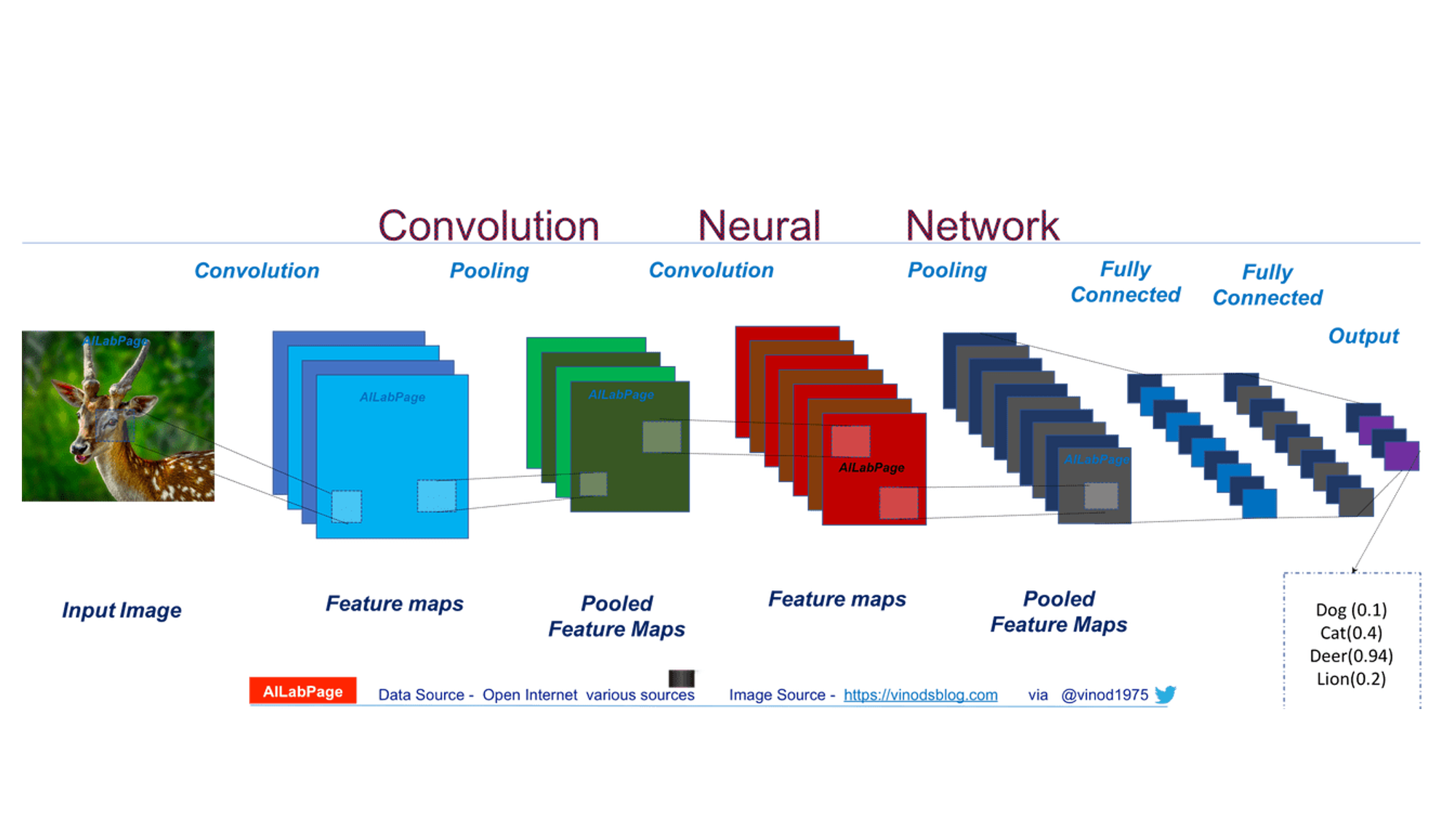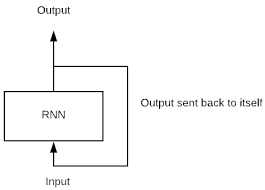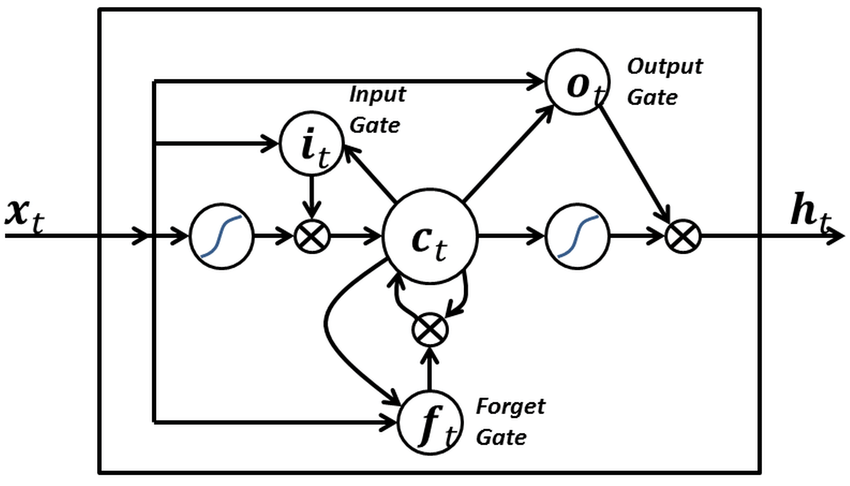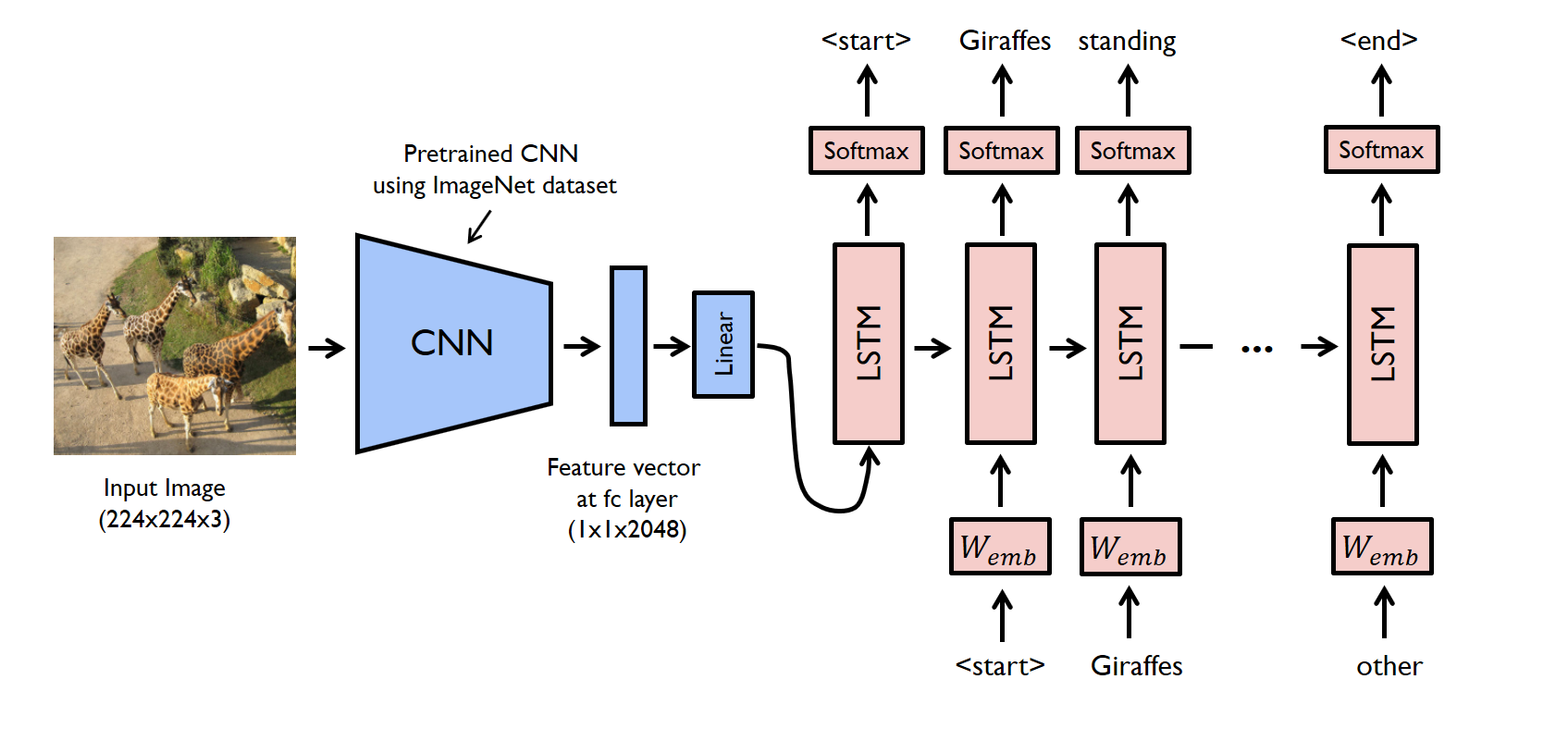×

Search anything:

# Differences between CNN and RNN

#### Machine Learning (ML)CNN (Convolution Neural Network) and RNN (Recurrent Neural Network) are two core Machine Learning models and are based on different fundamental ideas. In this article, we have explored the differences between CNN and RNN in depth.

## Introduction

Deep learning is a subfield of machine learning that deals with algorithms that are inspired from the structure, function and workings of the human brain. Artificial neural networks (ANN) are computing systems that are used in deep learning to enable machines to reason and make decisions like a human. As we’ll be dealing with such networks solely from a computing perspective, we will refer to them simply as neural networks.

Two extremely popular types of neural networks are the Convolutional Neural Network (CNN) and Recurrent Neural Network (RNN). Although CNNs and RNNs are both neural networks, they have different structures and applications. In this article, we'll be comparing the two networks.

## Differences between CNN & RNN

• CNN is a class of neural networks, generally used to analyze visual images. The network uses a mathematical operation called convolution which is a specialized kind of a linear operation. The hidden layers of a CNN usually consist of convolutional layers, ReLU layers, pooling layers, and fully connected layers.
RNNs are a type of Neural Network where the output from the previous node is an input to the current node, which is unlike traditional artificial neural networks in which the inputs and outputs are independent of each other. An important feature of RNN is the “hidden state” which remembers information about a sequence.

• The steps involved in training a CNN are:

• Convolutional layers apply a convolution operation to the input. This passes the information on to the next layer.
• Pooling combines the outputs of clusters of neurons into a single neuron in the next layer.
• Fully connected layers connect every neuron in one layer to every neuron in the next layer.

Each convolutional layer of a CNN contains a series of filters, called convolutional kernels. The filter is a matrix of integers that are used on a subset of the input pixel values, which are the same size as the kernel.
A diagram of a CNN that extracts image features is shown below• The steps involved in training an RNN are as follows:
• A single time step of the input is provided to the network. The current state is then computed using a set of the current input and the previous state. This current state becomes ht-1 for the next time step and so on.
• Once all the time steps are completed, the final current state is used to calculate the output.
• The output generated is then compared to the actual output i.e. the target output and the error is generated.
• The error is then back-propagated to the network to update the weights and hence the network (RNN) is trained. Thus, the error amount is divided among the connections.

A simple diagram is given below• In simple terms, a CNN is a feed forward neural network that applies a filter over the input signal to get a modified output signal. Feed forward neural networks are the simplest type of networks where the information moves ina single direction only; from the input nodes, to the hidden nodes (if any) and finally to the output layer. There are no loops or cycles in such a network. RNNs, unlike feed-forward neural networks, can "remember" through their internal memory to process sequences of inputs. The information is back-propagated to take into account previous information.

• CNNs are suitable for spatial data, such as images and is widely used in image and video processing. They can capture the spatial features, i.e. the arrangement and the relationships between the pixels in an image. This makes CNNs particularly useful in identifying objects, as well as their position within and in relation to other objects in an image. Since RNNs analyze the input sequentially and the output is returned to a previous layer (feedback loop), they are more suited to process temporal or sequential data, such as time series.

• While generating the output, CNNs consider only the current input while RNNs consider the current and previously received input (retains important information due to its internal memory). This makes RNNs ideal for text and speech analysis where previous inputs are required to be retained. However, in a simple RNN representation (such as the one show below), the network cannot remember important information that it may require later i.e. it cannot handle long term dependencies as the gradients which are back-propagated can "vanish" (i.e tend to zero) or "explode" (i.e., tend to infinity), as the computations involved use finite-precision numbers.
To overcome this problem, we use LSTM (long short term memory). LSTMs are a special type of RNNs that can selectively remember patterns for a long duration of time. In LSTMs, the information flows through a mechanism known as cell states. A common LSTM unit is composed of a cell, an input gate, an output gate and a forget gate.• CNNs takes only handle fixed size inputs and generate fixed size outputs whereas RNNs are able to handle arbitrary input/output lengths. This feature of RNNs is particularly useful while dealing with input sequences of text where the length of sentences often vary.

• Due to the nature of the network, as RNNs consider historical information, they are computationally slower. Therefore, CNNs are considered to be more powerful as they also have more feature compatibility than RNNs.

## CNN-RNN Hybrid Network

Despite their differences, as they both can perform classification of image & text inputs, CNNs & RNNs are not mutually exclusive and are often employed together to increase a network's effectiveness. A combination of the two networks (called a CRNN) is typically used when the input is visual with added temporal characteristics that a CNN alone would be unable to process. Such hybrid architectures have found applications in the fields of image captioning, emotion detection, video scene labelling and DNA sequence prediction.

An example of such an architecture being used for image captioning is given below.Hope this article at OpenGenus proved useful to you and you got the complete idea of the differences of CNN and RNN. Enjoy.